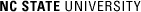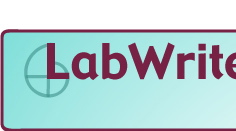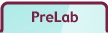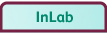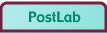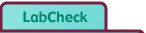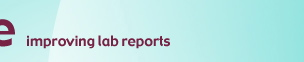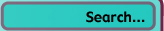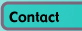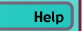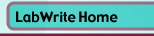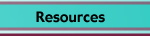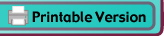# Designing ExperimentsSelfGuide

## Where do scientific experiments come from?

In some labs, you will be given a scientific problem to solve by designing your own experiment. If that is the case, go on to PreLab Question #1. But if you have been asked to come up with your own scientific problem, you need to do some thinking before beginning to answer the PreLab questions. For help in finding a scientific problem, click here.

## PreLab: questions to answer before doing the lab

1. What is the problem? Describe the problem in your own words. Be sure that your description includes known factors (information about the problem given to you in the lab in a problem statement, for example) and unknowns (what you need to find out in order to solve the problem). Then restate the problem in the form of a question or questions that will guide your research.

See example.

2. What do you know about the science of the problem that could help you answer your research question? State the scientific concept that the lab is about (something like the quantization of energy, photosynthesis, or momentum conservation). Write down information you can find about the concept that might be useful in answering your research question (check lab manual, textbook, class notes, handouts, etc.). Note any citations of sources you use. Go to Citations and References for more help.

See example.

3. What is your hypothesis for the answer to your research question? Using what you know about the problem and the scientific concept of the lab, state a hypothesis, your best estimation of the answer to your research question. Then describe the reasoning that led you to your hypothesis, using what you know about the scientific concept as a basis for your reasoning.

See example.

4. What variables can you use to test your hypothesis? A well-designed experiment needs to have variables. Look over your hypothesis, and identify the variables that you will be testing during your experiment: what you can measure or observe (dependent variables) and what you can manipulate in an experiment for the measurements or observations (independent variables). List your variables. Then describe, in words or in a sketch, the relationship among the variables as predicted by the hypothesis.

See example.

5. What experiment(s) could you use to test your hypothesis? Referring to the list of variables, brainstorm some experiments you could do that would allow you to manipulate variables so that you can make the measurements or observations necessary for testing the hypothesis.Your experiment may require control and treatment groups. Choose the experiment most likely to yield the results you need to test your hypothesis. List the materials and outline the methods you will use for your experiment. (Remember that you have to work with the materials and lab instruments available to you.)

See example.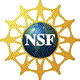© Copyright NC State University 2004 Sponsored and funded by National Science Foundation (DUE-9950405 and DUE-0231086) Site design by Rosa Wallace Rev. MF 6/6/05